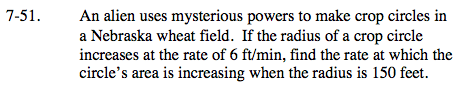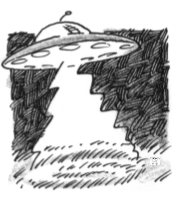### Home > CALC > Chapter 7 > Lesson 7.1.5 > Problem7-51

7-51.

An alien uses mysterious powers to make crop circles in a Nebraska wheat field. If the radius of a crop circle increases at the rate of 6 ft/min, find the rate at which the circle's area is increasing when the radius is 150 feet. Homework Help ✎Start with the geometric equation of the area within a circle. There is no Calculus involved in this step.
A = πr²
Do NOT substitute a value for r, because the r changes. That is where Calculus comes in...Implicitly differentiate with respect to time, t.